Views from CastaliaThe title of this talk comes from the novel "The Glass Bead Game" by Hermann Hesse. The setting of the story is a serene location in the mountains called Castalia. There are three mountains (the preprojective variety, the quiver variety and the loop Grassmannian). These three mountains feed the spring at their base (the quantum group). At the spring live three nymphs, very similar, but different (the semicanonical basis, the canonical basis and the MV basis). Each of these is nourished by the special nutrients of the corresponding source (the preprojective variety, the quiver variety and the loop Grassmannian). But the shadow of the muses is the same (the MV polytope). And, as we shall see, there is a Glass Bead Game in this story as well.

Thank you

This is a lecture given at the IBS Symposium and Meeting in Seoul Korea on 7 August 2013. Thank you to IBS, for the invitation and to the team who has organised so many details in making the arrangements perfect.

The images of the IAS seal are from

• http://cs.cinvestav.mx/~gmorales/teatro/images/SealIAS.png
• http://www.ias.edu/news/press-releases/2013/03/10/ias-seal
• from a google image search claiming this is on the page http://video.ias.edu/0405, though I didn't find it there myself
Thank you to the IAS for permission to use the image of the seal in this presentation.

Other images are mostly from Wikimedia Commons distributable by the GNU license from the following sources. Thanks to many contributors for these excellent resources.

. . . and thank you to my collaborators Alex Ghitza (Melbourne) and Senthamarai Kannan (Chennai) for all their contribution to the ideas being pursued in this talk.

The three mountains: The preprojective variety, the quiver variety and the loop Grassmannian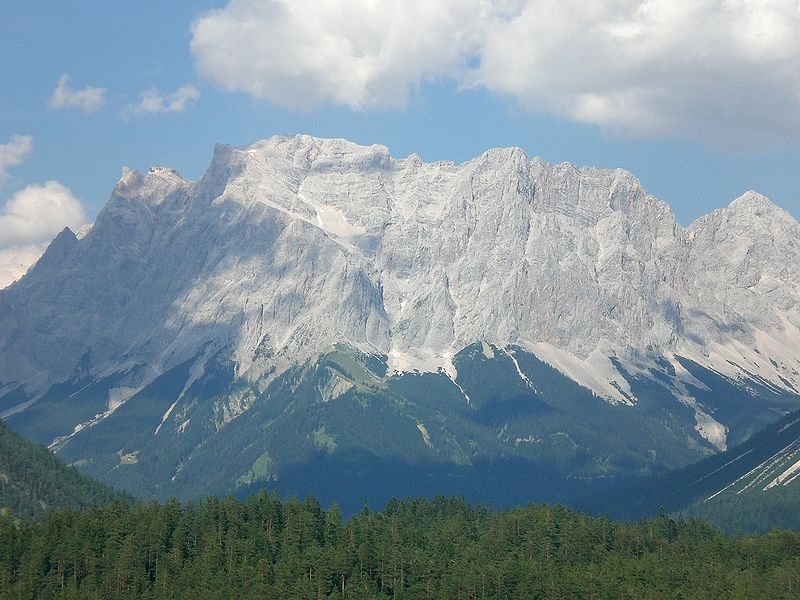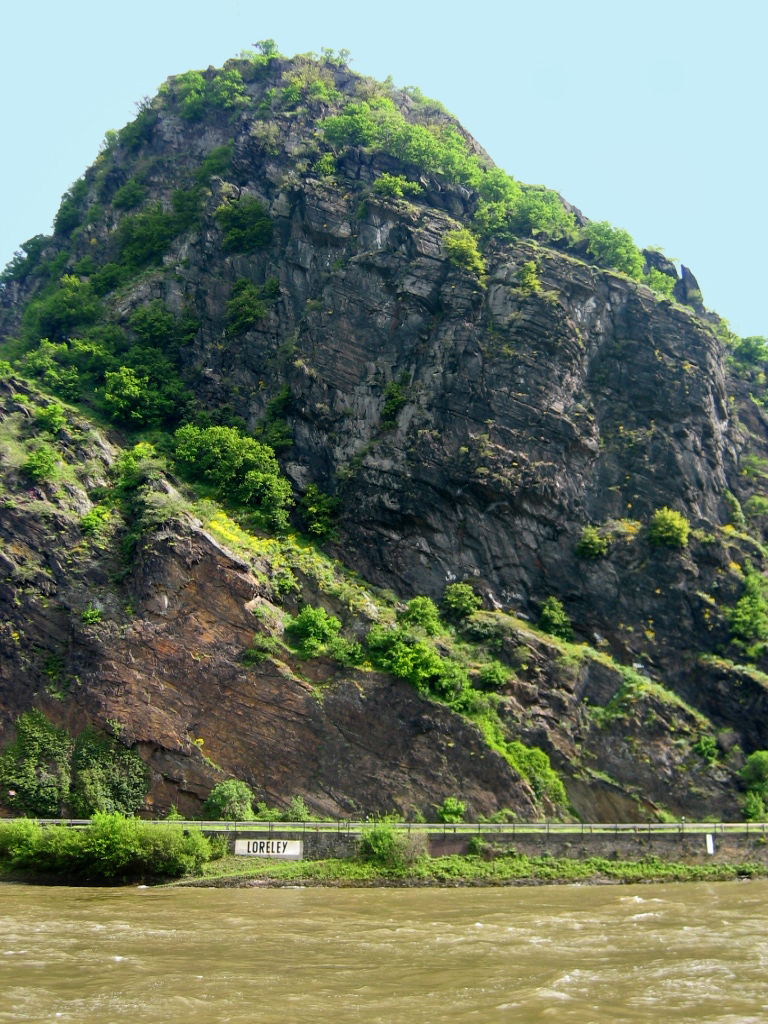Preprojective variety Quiver variety or KLR modules Loop Grassmannian $G$ Irreducible components ${\Lambda }_{b}$ Irreducible modules ${L}_{b}$ MV-cycles ${Z}_{b}$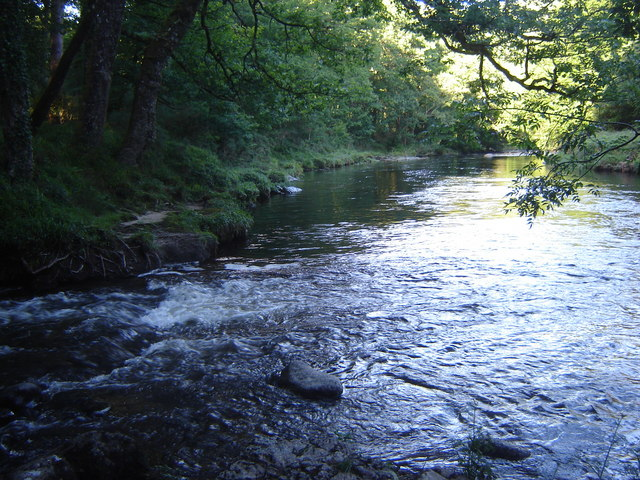The character map
 The Quantum group ${U}_{q}{𝔫}^{-}$$\mathrm{char}\left({\Lambda }_{b}\right)$ The semicanonical basis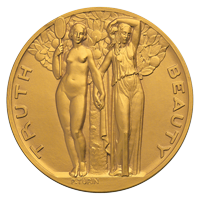$\mathrm{char}\left({Z}_{b}\right)$ The MV basis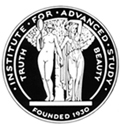$\mathrm{char}\left({L}_{b}\right)$
The canonical basis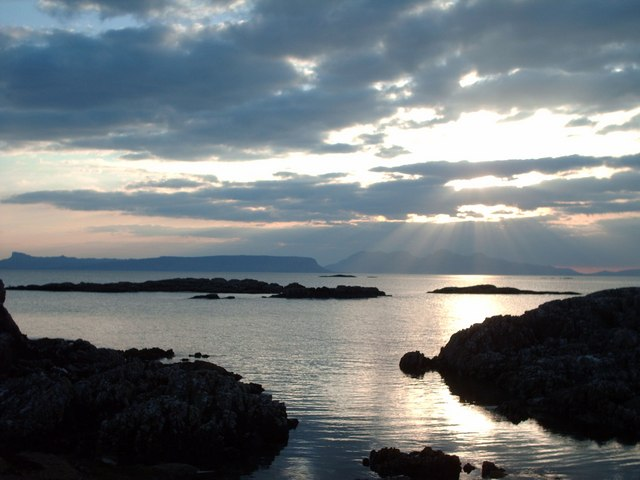The shadow map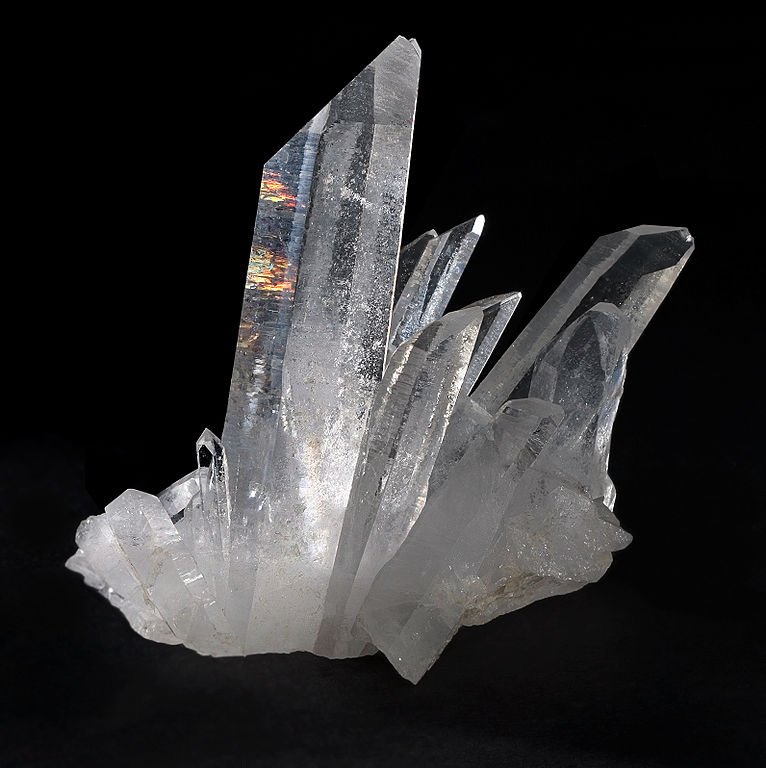MV polytopes: The crystal
$\mathrm{shad}\left(\mathrm{char}\left({\Lambda }_{b}\right)\right)=\mathrm{shad}\left(\mathrm{char}\left({L}_{b}\right)\right)=\mathrm{shad}\left(\mathrm{char}\left({Z}_{b}\right)\right)=b$

The crystal shadow is exactly the same for all three mountains:
The preprojective variety, the quiver variety and the loop Grassmannian.

The loop Grassmannian G/K

$ℂ((t)) = { aℓt-ℓ+ aℓ+1 t-ℓ+1+… ∣ai ∈ℂ,ℓ∈ℤ } ⊆ ℂ[[t]] = { a0+a1t+ a2t2+… ∣ai∈ℂ } ⟶t=0 ℂ.$

$G\left(ℂ\right)$ is a complex reductive algebraic group, say $G={\mathrm{PGL}}_{3}\text{.}$

$G= PGL3 (ℂ((t))) ⊆ K= PGL3 (ℂ[[t]]) ⟶t=0 PGL3(ℂ)$

$G}{K}$ is the loop Grassmannian, or affine Grassmannian.

The loop Grassmannian is studied with the decompositions $G=⨆λ∈𝔥ℤ+ KtλKand G=⨆μ∈𝔥ℤ U-tμK$ where $tλ= ( t -λ1 0 0 0 t -λ2 0 0 0 t -λ3 ) andU-= { ( 10 ⋱ ✶1 ) ∣✶∈ ℂ((t)) } .$

The Mirkovic-Vilonen intersections are $KtλK∩U- tμK.$ The MV cycles are the irreducible components $Zbin Irr ( KtλK∩ U-tμK ‾ ) .$

Dynkin diagrams

Let ${G}^{\vee }$ be the Langlands dual complex reductive algebraic group. In our example, $G∨ = SL3(ℂ) has a maximal torus T∨ = { ( x1 0 0 0 x2 0 0 0 x3 ) with x1, x2, x3∈ ℂ× and x1 x2 x3 =1 }$ The Weyl group and the character lattice are $W0= N(T∨) T∨ and 𝔥ℤ= Hom( T∨,ℂ× ),$

In our example, $W0= ⟨ s1, s2 | si2=1, s1 s2 s1 = s2 s1 s2 ⟩ and 𝔥ℤ= ℤspan{ ω1, ω2 }.$ $ω1 ω2 𝔥α1∨ 𝔥α2∨ C0 s1C0 s2C0 s1s2C0 s2s1C0 s1s2s1C0=s2s1s2C0$

Parallel lines of lattice points $𝔥α1∨ 𝔥α2∨ C0 s1C0 s2C0 s1s2C0 s2s1C0 s1s2s1C0=s2s1s2C0 𝔥α1∨ 𝔥α2∨ C0 s1C0 s2C0 s1s2C0 s2s1C0 s1s2s1C0=s2s1s2C0$ produce $𝔥α1∨ 𝔥α2∨ C0 s1C0 s2C0 s1s2C0 s2s1C0 s1s2s1C0=s2s1s2C0$ with fundamental alcove (contained in ${C}_{0}$ and adjacent to the origin) $𝔥α1∨ 𝔥α2∨ 𝔥α0∨ C0 s1C0 s2C0 s1s2C0 s2s1C0 s1s2s1C0=s2s1s2C0$ The fundamental alcove has walls ${𝔥}^{{\alpha }_{1}^{\vee }},{𝔥}^{{\alpha }_{2}^{\vee }}$ and ${𝔥}^{{\alpha }_{0}^{\vee }}$, $C0 𝔥α1∨ 𝔥α2∨ 𝔥α0∨ π3$ and the extended Dynkin diagram, or affine Dynkin diagram, is the dual graph of the fundamental alcove. This means: Make a graph with vertices $0,1,\dots ,n$ and $i j i j i j i j if𝔥αi ∡𝔥αjis π2 π3 π4 π6$

In our example, the $S{L}_{3}$ case: $C0 𝔥α1∨ 𝔥α2∨ 𝔥α0∨ π3 0 1 2 gives 0 1 2$

KLR Quiver Hecke algebras     $R=\underset{d\in {ℤ}_{\ge 0}}{⨁}{R}_{d}$

$I=vertex set of Dynkin diagram= {colours}.$

The KLR quiver Hecke algebra ${R}_{d}$ is given by generators $y1,…,yd, euforu∈Id, ψ1,…,ψd-1$ with relations $yiyj =yjyi , euev =δuv eu , euyi =yieu , euψr =ψr esru , 1= ∑u∈Id eu, ψryi=yiψr ifi≠r,r+1, ψryreu= { (yr+1ψr+1)eu , if(ur,ur+1) =(ur,ur) , yr+1ψreu , otherwise. ψryr+1eu= { (yrψr-1)eu , if(ur,ur+1) =(ur,ur) , yrψreu , otherwise. ψrψs=ψsψr, ifs≠r,r±1, ψr2eu= { 0, if(ur,ur+1) =(ur,ur) , (yr+1-yr)eu , if(ur,ur+1) is ur ur+1 , -(yr+1-yr)eu , if(ur,ur+1) is ur ur+1 , eu , otherwise. ψrψr+1ψr eu= { ( ψr+1 ψr ψr+1 +1 ) eu, if ( ur,ur+1, ur+2 ) = ( ur,ur+1, ur ) with ur ur+1 , ( ψr+1 ψr ψr+1 -1 ) eu, if ( ur,ur+1, ur+2 ) = ( ur,ur+1, ur ) with ur ur+1 , ψr+1 ψr ψr+1 eu , otherwise. yiyj =yjyi , euev =δuv eu , euyi =yieu , euψr =ψr esru , and more ...$ where $Id= { u= (u1,…,ud) sequences of length d inI } and sru isu with ur and ur+1switched.$

. . .     and ${R}_{d}$ has $ℤ$-grading $deg (eu) =0 , deg(yi)=2, deg(ψreu)= { -2, ifur= ur+1 -1, if ur ur+1 0, if ur ur+1$

Let $Mbe a ℤ-graded R -moduleso that M=⨁j∈ℤ M[j]$ Define $char(M)= ∑j∈ℤ ∑u∈Id dim(euM[J]) qjfu1… fud.$

(generating function in noncommutative ${f}_{i},\phantom{\rule{0.2em}{0ex}}i\in I\text{).}$

(Khovanov-Lauda, Rouquier) $char: Grothendieck group of { fin. dim'lℤ-graded Rmodules } ⟶ Uq𝔫- (quantum group) simple R-modules Lb ⟼ char(Lb) (canonical basis)$

$I=vertex set of Dynkin diagram= {colours}.$

$Board Beads$

A skew shape is a configuration of beads $\lambda$ such that any two beads on the same runner are separated by two beads. $if then or$

A standard tableau of shape $\lambda$ is a runner sequence $T=\left({T}_{1},\dots ,{T}_{d}\right)$ which results in $\lambda$. For example, $1 4 2 3 is (g,y, r,g) and 1 4 3 2 is (g,r, y,g)$

Define $Lλ =span { vT∣T is a standard tableau of shapeλ } with$ $euvT= δuTvT, yivT=0, ψrvT= { vsrT, ifsrT is a standard shape, 0, otherwise.$

(Kleshchev-Ram) The ${L}_{\lambda }$ are simple ${R}_{d}$-modules.

Preprojective algebras

$Q= Q‾=$

Idea: Replace beads by vector spaces.

$ℂn1 ℂn2 ℂn3 ℂnj corresponds to njbeads on runnerj.$

The data of

 a vector space for each vertex a linear transformation for each edge $\phantom{\rule{5em}{0ex}}$ is a representation of the quiver.
Then $Λ= ∏edges M ni nj (ℂ) ∼ (quiver variety and preprojective variety)$ where $\sim$ is equivalence of representations (change of basis in the vector spaces).
In the case of $\stackrel{‾}{Q}$ also require $∑ i a ∈Q a*a= ∑ i a ∈Q aa*,for eachi∈I.$

Then ${\Lambda }_{b}$ are the irreducible components of $\Lambda$.

PhilosophyPreprojective variety $\Lambda$ Quiver variety $X$ Loop Grassmannian $G/K$ $G$ Irreducible components ${\Lambda }_{b}$ Irreducible modules ${L}_{b}$ MV-cycles ${Z}_{b}$ Functions/Sheaves/Orbit convolution on Functions/Sheaves/Orbit convolution on Functions/Sheaves/Orbit convolution on Cotangent bundle $\Lambda ={T}^{*}\left(X\right)$ $X$ $G/K\phantom{\rule{0.2em}{0ex}}\cap \phantom{\rule{0.2em}{0ex}}{U}^{-}\G$ with character ring $U{𝔫}^{-}$ with character ring ${U}_{q}{𝔫}^{-}$ with character ring $ℂ\left[{N}^{-}\right]$

The idea of the relationship between these is $sheaves on Λ= T*(X) sheaves onX sheaves on G/K∩ U-\G exponential map characteristic variety or singular support$

Thank you.